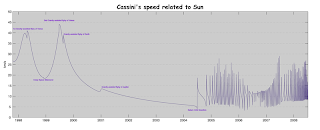## Wednesday, November 13, 2013

### Capstones! Gravitational Slingshot edition

My AP class does capstone projects at the end of each term. It's a short independent project, having to do with anything from the term, which they execute and present in such a way that we can post them here.

Here's the first of the crop of this fall's capstones: a VPython project simulating a gravity assist.

Student work:
The goal of my Capstone project was to create a program in VPython which simulates the gravitational slingshot used by satellites such as Voyager I or Cassini.  This method, officially called “gravity assist”, is used by space programs such as NASA to send probes to distant targets without draining resources since it uses the natural gravitational forces as ways to propel the probes into space.

In the program, I send a 15,000 kg probe into orbit around the Earth while also having the Moon orbit the Earth.  By adjusting the initial velocity of the probe, the probe would be able to pass by the moon and use the Moon’s gravitational force to “slingshot” it off to a “target” asteroid away from the Earth.  Finally, I graphed the speed of the probe during its journey and compared it to the velocity graph of Cassini.  The small boost in the graphs shows the moment the probe uses the gravitational slingshot, similar to the Cassini graph when it orbits around Venus.

Images:Cassini's speed graph (wikipedia)The program, after probe has made it to the targetv graph from the program, showing the boost

Cassini Graph citation:

"Cassini's Speed Related to the Sun." Chart. Wikipedia. Wikimedia, n.d. Web. 12 Nov. 2013. .

Code (syntax highlighting finally works!):
    from __future__ import division
from visual.graph import*
from visual import*

#Richie Lou
#Gravitational Slingshot - CAPSTONE

#OBJECTIVE: to use a gravitational force to send a space shuttle from Earth's orbit
#to a target asteroid by using the moon as a gravitational slingshot

#Create Shuttle
Shuttle=box(pos=(6.4e7,0,0), length=72.8, width=108.5, height=20, color=color.red, make_trail=True)
Shuttle.m=15000 #kg
Shuttle.v=vector(0,-3350,0) #m/s

#Create Earth
Earth.m=6e24 #kg

#Create Moon
Moon=sphere(pos=vector(0,4e8,0), radius=1.75e6, color=color.white, make_trail=True)
Moon.m=7e22 #kg
Moon.v=vector(1050,0,0) #m/s

#Create Target Asteroid
Target=sphere(pos=vector(-1.40837e9, 1.42004e9, 0), radius=7e6, color=color.green)

#Create Initial Conditions
G=6.67e-11 #N*(m/kg)^2 #Gravitational Constant

R=Shuttle.pos-Moon.pos #m
r=Shuttle.pos-Earth.pos #m

M=Moon.pos-Earth.pos #m

F=Shuttle.pos-Target.pos #m

FnetShuttle=-(G*Earth.m*Shuttle.m*r)/(mag(r)**3)-(G*Moon.m*Shuttle.m*R/(mag(R)**3)) #N
FnetMoon=-(G*Earth.m*Moon.m*M)/(mag(M)**3)+(G*Moon.m*Shuttle.m*R/(mag(R)**3)) #N

deltat=50 #s
t=0 #s

#Graph Velocity
gdisplay(x=0, y=0, width=600, height=150, title="velocity vs. time", xtitle="t", ytitle="velocity (m/s)", foreground=color.black, background=color.white)
g=gcurve(color.red)

#Animate Orbit
while mag(R) > 1.75e6 and mag(r) > 6.4e6 and mag(F) > 7e6:
Shuttle.pos=Shuttle.pos+Shuttle.v*deltat #m #position update
Shuttle.v=Shuttle.v+(FnetShuttle/Shuttle.m)*deltat #m/s #velocity update

Moon.pos=Moon.pos+Moon.v*deltat #m #position update
Moon.v=Moon.v+(FnetMoon/Moon.m)*deltat #m/s #velocity update

R=Shuttle.pos-Moon.pos #m
r=Shuttle.pos-Earth.pos #m

M=Moon.pos-Earth.pos #m

F=Shuttle.pos-Target.pos #m

FnetShuttle=-(G*Earth.m*Shuttle.m*r)/(mag(r)**3)-(G*Moon.m*Shuttle.m*R/(mag(R)**3)) #N #Force update
FnetMoon=-(G*Earth.m*Moon.m*M)/(mag(M)**3)+(G*Moon.m*Shuttle.m*R/(mag(R)**3)) #N #Force update

t=t+deltat #s #time update

rate(1e100)

g.plot(pos=(t,mag(Shuttle.v)))

print t/8.64e4, "days"
print mag(Shuttle.v), "m/s"